## Max Points on a Line

Given n points on a 2D plane, find the maximum number of points that lie on the same straight line.

### Analysis:

First of all, how to know the points are on the same line?  The slope!
The slope of a line can be computed using any two points (x1,y1) (x2,y2) on the line:
slop = (y2-y1)/(x2-x1) , x2!=x1.

In this problem, we want to know the max number of points on one line. We can think in this way: for every single point, all the possible lines (slopes) which goes across this point and any other point in the plane can be easily computed. Why? Because at least two points can determine a line, if one point is now fixed, use every other point can get all the lines.  For all these lines, they have the same starting point, so if two lines La--Lb and La--Lc have same slope, points a,b and c are actually on the same line. See figure below:

So, we can have a loop from the 1st point to the last point. Within this loop, we compute the slopes from this point to every other point. Then find the max number of points, which has the same slope. e.g., in the figure above, for point 1 (p1), the slops computed are
S:[s11,s12,s13,s14,s15,s16].
Say, s11 to s16 are six lines connecting p1 and p2,3,4,5,6 respectively. If s1i==s1j, i!=j, then p1, pi and pj are on the same line. The maximum number of points on the same line here in this one iteration is the max number of same slopes in S. Once we have this number in one iteration, the max number of all the iterations is what we want.

The algorithm goes like this:
%%%%%%%%%%%%%%%%%%%%%%%%%%%%%
for each point i in the plane:
for each point j in the plane:
if i!=j,
Compute and store the slop
Sort the slop array
Count how many slops are the same
Store the max number

Output the max number
%%%%%%%%%%%%%%%%%%%%%%%%%%%%%

Note: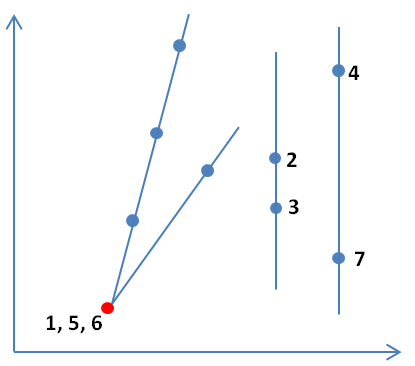(1) The slop is computed using "/", if (x2-x1)==0, there will be an error, but in the 2D plane it is a valid line (vertical line). So, store the vertical line as a specific slope is needed.

(2) Remember to consider the input points have duplicates. In my code, I only consider the duplicates with the starting point in each loop, the slop is not computed then but the number of duplicates are stored instead. Also be careful with all the input points are the same case.

See code for more details.

The complexity of this problem is :   O(n*nlgn).    n is the big loop i, nlgn is the sorting complexity (O(nlgn)>O(n))

### Code(C++):

/**
* Definition for a point.
* struct Point {
*     int x;
*     int y;
*     Point() : x(0), y(0) {}
*     Point(int a, int b) : x(a), y(b) {}
* };
*/
class Solution {
public:
int maxPoints(vector<Point> &points) {
int n = points.size(); //number of the points
if (n<=2){return n;}
vector<double> k; //vector to store the slops for one point with all the other points
int res = 0;

for (int i=0;i<n;i++){ // for each point in the 2d plane
k.clear();
int dup = 1; // number of the duplicates with currrent point
for (int j=0;j<n;j++){
if (i!=j){ // for every other point
if (points[i].x-points[j].x==0){ // if the slope is a vertical line
if (points[i].y-points[j].y==0){ // if the point j is the same as point i
dup++;
}else{
k.push_back(99999); //store the vertical line to a specific slope
}
}else{ // if it is the regular slop between point i and j
k.push_back(10000*(points[i].y-points[j].y)/(points[i].x-points[j].x)); // store the slope
}
}
}

sort(k.begin(),k.end()); //sort the slopes for counting

int pp = 1; //number of points in the same line of the point i
if (k.size()==0){pp=0;}

for (int jj=1;jj<k.size();jj++){ //count pp
if (k[jj]==k[jj-1]){
pp++;
}else{
if (pp+dup>res){res=pp+dup;} // res = pp + the number of duplicates
pp = 1;
}
}
if (pp+dup>res){res = pp+dup;}
}

return res;
}
};

### Code(Python):

# Definition for a point
# class Point:
#     def __init__(self, a=0, b=0):
#         self.x = a
#         self.y = b

class Solution:
# @param points, a list of Points
# @return an integer
def maxPoints(self, points):
if (len(points)<=2):
return len(points)
m = 0 #result value
for i in range(0, len(points)):
l = {}  #every time reset the dict
dup = 1 #count the duplicates
for j in range(0, len(points)):
if (points[i].x==points[j].x and points[i].y==points[j].y and i!=j):
dup+=1  #count duplicates
elif i!=j :
if (points[i].x==points[j].x):  #vertical line
l['v'] = l.get('v',0) + 1
elif (points[i].y==points[j].y): # horizontal line
l['h'] = l.get('h',0) + 1
else:   #regular slope
slope = 1.0*(points[i].y-points[j].y)/(points[i].x-points[j].x)
l[slope] = l.get(slope,0)+1
if (len(l)>0):
m = max(m,max(l.values())+dup)
else: #if all points are duplicates, l is empty.
m = max(m,dup)
return m



1.Slopes of different parallel line would be same, but that does not mean that they lie on the same line. How are you tackling this issue ?

1.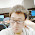When compute the slopes for each point, the lines are all started from the same point, and connected to other points, the counting of same slopes is under this condition. The parallel line have the same slope but never have the same point. I've modified the description of the analysis part, please check it out. Thanks.

2.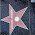This comment has been removed by the author.

3.Thank you for your great blog, I have learned a lot.
I have a question for this sentence at line 30:
(points[i].y-points[j].y)/(points[i].x-points[j].x)
My question is does it have precision truncation issues? I mean, precision truncated before assign to the double precision number?

4.5.如果用hash来装slope, 就不用sort了，可以把 running time 从 n*nlogn 降到 n*n

public int maxPoints(Point[] points) {
if (points.length <= 1 ) return points.length;
int max = 0;
int kVerticalSlope = Integer.MAX_VALUE; // speicial constant value
for (int i=0; i slopeMap = new HashMap();
Point p0 = points[i];
int duplicate = 0;
for (int j = 0; j max) {
max = num;
}
}
}
return max;
}

这个缺点就是要用double值来做hash的key，不过面试的时候你说给面试官听，你注意到这点并提供口头solution，就行了

6.很悲催，代码贴不出来，反正我的意思是用一个 slope->num of this slope 的hash

1.Yes, definitely you can use the hash.
Is it the same meaning as my python code?

7.Can we say that line(p1,p2) and line(p1,p3) are in the same line if slope(p1,p2) *10000 = slope(p1,p3)*10000 ?There might be possible that line(p1,p2) is very very closed to line(p1,p3) but they are not in the same line. Slope*10000 might have some precision problem.

8.Hi ,
There are two for loops in the solution, How can it be O(n log n) , I guess its O(n*n) right. Can anyone please explain

Guru

1.In my code, the complexity is O(n * n * logn), which is O(n^2 logn),

9.1. why multiply 10000? if the slope itself is over 9, it'd be close to 99999 as the vertical
2. Aren't duplicates the same as verticals? if x difference is 0, how about just assign INFINITY to slope?

Thanks

1.Sorry, duplicates are not the same as verticals. But assigning INFINITY works.

Still, for multiplication, if I do
10000*( (points[j].y-points[i].y)/(points[j].x-points[i].x)) )
it doesn't work, always missing points

but your way works fine.
Why do we have to do multipication before division?

Also, the number has to be at least 10000, 1000 doesn't work (tried :()

10.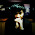Good solution. Some suggestions: 1. use map to count the same slop will avoid sort. 2. slop is float, is it a good way to use "=="? 3. You actually don't need to compute the slop for every two points multiple times. The outer loop (int i) goes from the first to the last node and the inner loop actually can go from the ith node to the last one.

11.For the inner loop, you don't have to start from 0, instead you can start from i+1, which can saves some time.

12.This comment has been removed by the author.

13.each line is ax+by+c=0
simply use [a,b,c] as key and the set of points on that line as value into a hashmap,
each time with pi and pj, just calculate out a b c and insert/update the points set , and also update the max
when a points set changed.

14.Thanks for your blog! I have a question.
In the inner for loop, why does j begins with 0 instead of i+1?
I think j begins with 0 will also get the same res value in your code. Because for a line containing the most points, there is always a point traversed in the outer loop and other points in this line traversed in the inner loop. Am I right?

15.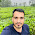Another solution :

/**
* Definition for a point.
* struct Point {
* int x;
* int y;
* Point() : x(0), y(0) {}
* Point(int a, int b) : x(a), y(b) {}
* };
*/
class Solution {
public:

int gcd(int a,int b){
if(!b) return a;
return gcd(b,a%b);
}

void setSign(int &x,int &y){
if(x>0 && y>0) return;
if((x<0 && y<0) || (x>0 && y<0)){
x=-x;
y=-y;
return;
}

if(x==0){
if(y<0) y=-y;
return;
}
if(y==0){
if(x<0) x=-x;
return;
}
}

int maxPoints(vector &points) {
map ,int > slope;
map, int > ::iterator it;
int sum=0,ans=0;
for(int i=0;i p=make_pair(x,y);
it=slope.find(p);
if(it!=slope.end())
it->second++;
else
slope[p]=1;
}
else
dup++;
}
}
sum=0;
for(it=slope.begin();it!=slope.end();it++){
if(it->second > sum)
sum=it->second;
}
sum+=dup;
if(sum>ans)
ans=sum;
}
return ans;
}
};

16.有点优化，第一个嵌套循环里 j 可以直接从i+1 开始，这样要快不少虽然还是n*n.因为包含points~points[i]的点，之前已经考虑过了。

17.Will the time complexity be n^2 since for every element, you go through n-1 comparisons, and there are n elements in total, so that's n^2...